# RS Aggarwal Class 9 Solutions Chapter 2 Polynomials Ex 2B

## RS Aggarwal Class 9 Solutions Chapter 2 Polynomials Ex 2B

These Solutions are part of RS Aggarwal Solutions Class 9. Here we have given RS Aggarwal Solutions Class 9 Chapter 2 Polynomials Ex 2B.

Other Exercises

Question 1.
Solution:
p(x) = 5 – 4x + 2x2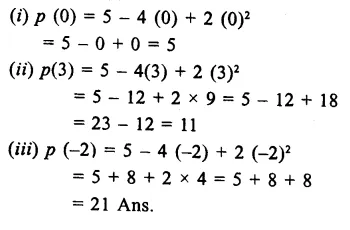Question 2.
Solution:
p(y) = 4 + 3y – y2 + 5y3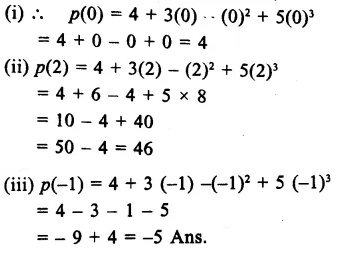Question 3.
Solution:
f(t)=4t2-3t+6Question 4.
Solution: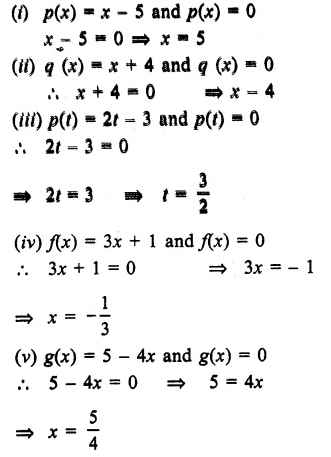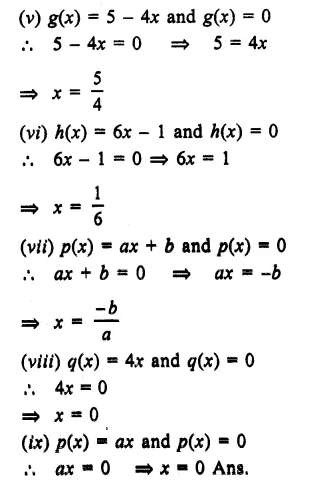Question 5.
Solution: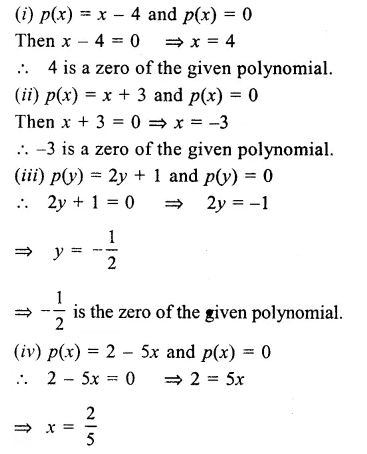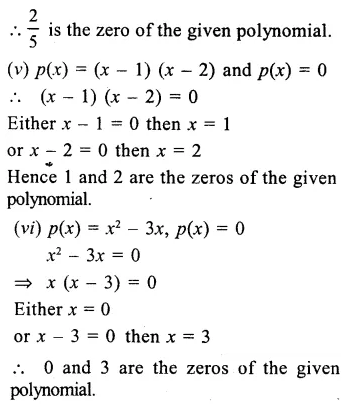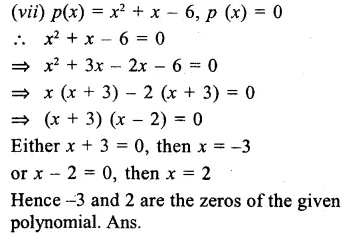Hope given RS Aggarwal Solutions Class 9 Chapter 2 Polynomials Ex 2B are helpful to complete your math homework.

If you have any doubts, please comment below. Learn Insta try to provide online math tutoring for you.